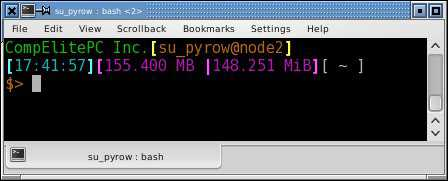# Customizing BASH: PS1

June 2nd, 2007 By: SU_PYROW

There are allot of white papers on customizing the bash prompt. This one is of course different... by a small margin. It starts by mapping out colors from "\033" or "ESC" commands into text commands for better reading, modifying, or if you change your background with a transparent terminal running most of the time you need to change colors of your text, this should help somewhat.

You will need a dependency:

```#.bashrc
DULL=0
BRIGHT=1
FG_BLACK=30
FG_RED=31
FG_GREEN=32
FG_YELLOW=33
FG_BLUE=34
FG_VIOLET=35
FG_CYAN=36
FG_WHITE=37
FG_NULL=00
BG_BLACK=40
BG_RED=41
BG_GREEN=42
BG_YELLOW=43
BG_BLUE=44
BG_VIOLET=45
BG_CYAN=46
BG_WHITE=47
BG_NULL=00

ESC="\033"
NORMAL="\[\$ESC[m\]"
RESET="\[\$ESC[\${DULL};\${FG_WHITE};\${BG_NULL}m\]"
BLACK="\[\$ESC[\${DULL};\${FG_BLACK}m\]"
RED="\[\$ESC[\${DULL};\${FG_RED}m\]"
GREEN="\[\$ESC[\${DULL};\${FG_GREEN}m\]"
YELLOW="\[\$ESC[\${DULL};\${FG_YELLOW}m\]"
BLUE="\[\$ESC[\${DULL};\${FG_BLUE}m\]"
VIOLET="\[\$ESC[\${DULL};\${FG_VIOLET}m\]"
CYAN="\[\$ESC[\${DULL};\${FG_CYAN}m\]"
WHITE="\[\$ESC[\${DULL};\${FG_WHITE}m\]"
BRIGHT_BLACK="\[\$ESC[\${BRIGHT};\${FG_BLACK}m\]"
BRIGHT_RED="\[\$ESC[\${BRIGHT};\${FG_RED}m\]"
BRIGHT_GREEN="\[\$ESC[\${BRIGHT};\${FG_GREEN}m\]"
BRIGHT_YELLOW="\[\$ESC[\${BRIGHT};\${FG_YELLOW}m\]"
BRIGHT_BLUE="\[\$ESC[\${BRIGHT};\${FG_BLUE}m\]"
BRIGHT_VIOLET="\[\$ESC[\${BRIGHT};\${FG_VIOLET}m\]"
BRIGHT_CYAN="\[\$ESC[\${BRIGHT};\${FG_CYAN}m\]"
BRIGHT_WHITE="\[\$ESC[\${BRIGHT};\${BG_WHITE}m\]"
REV_CYAN="\[\$ESC[\${DULL};\${BG_WHITE};\${BG_CYAN}m\]"
REV_RED="\[\$ESC[\${DULL};\${FG_YELLOW}; \${BG_RED}m\]"

PROMPT_COMMAND='export ERR=\$?'
```

Its possible you need a ".bash_profile" here is one you can use if you don't have one. Put it in your home directory, along with ".bashrc"

```#.bash_profile
source .bashrc
if [ -f ~/.bashrc ]; then
. ~/.bashrc
fi
#End Script
```

Lets put it to work: I personally would comment out my currently working ##"PS1=" and put it below it in case you need to change it back for some reason.

Note: Works best if it is one line.

```PS1="\${GREEN}YOUR COMPANY NAME HERE\${BRIGHT_YELLOW}[\${YELLOW}\u@\h\${BRIGHT_YELLOW}]\n\${BRIGHT_CYAN}[\${CYAN}\t\${BRIGHT_CYAN}]\${BRIGHT_VIOLET}[\${VIOLET}\\$(~/sbin/lsMB)\${BRIGHT_VIOLET} | \${VIOLET}\\$(~/sbin/lsMiB)\${BRIGHT_VIOLET}]\${WHITE}[ \w ]\n\${YELLOW}\$> \${RESET}"
```

Should look like this minus the colors:

```YOUR COMPANY NAME HERE[su_pyrow@CompElitePC.host56.com]
[13:57:23][590.478 MB|563.316 MiB][/media/cdrom]
\$>
```

With colors:To get the sum of files in current working directory, I made two files which do the summing. "lsMB" and "lsMiB" which on my system are both located in ~/sbin as executable. Here is lsMB:

```#!/bin/bash
#lsMB free for anyone who wishes to implement it.
let TotalBytes=0
for Bytes in \$(ls -l -a | grep "^-" | awk '{ print \$5 }')
do
let TotalBytes=\$TotalBytes+\$Bytes
done
if [ \$TotalBytes -lt 1024 ]; then
TotalSize=\$(echo -e "scale=3 \n\$TotalBytes \nquit" | bc)
suffix="B"
else if [ \$TotalBytes -lt 1048576 ]; then
TotalSize=\$(echo -e "scale=3 \n\$TotalBytes/1024 \nquit" | bc)
suffix="kB"
else if [ \$TotalBytes -lt 1073741824 ]; then
TotalSize=\$(echo -e "scale=3 \n\$TotalBytes/1048576 \nquit" | bc)
suffix="MB"
else if [ \$TotalBytes -lt 1099511627776 ]; then
TotalSize=\$(echo -e "scale=3 \n\$TotalBytes/1073741824 \nquit" | bc)
suffix="GB"
fi
fi
fi
fi
echo -n "\$TotalSize \$suffix"
#End Script
```

Finally, this is the lsMiB executable file located in "~/sbin" This has the modification for the conversion to KiB, MiB & GiB one could further the code to handle TB/TiB easily by calculating from the above and using WIKI Terabyte and WIKI Tebibyte.

```#!/bin/bash
#lsMiB free for anyone who wishes to implement it.
let TotalBytes=0
for Bytes in \$(ls -l -a | grep "^-" | awk '{ print \$5 }')
do
let TotalBytes=\$TotalBytes+\$Bytes
done
if [ \$TotalBytes -lt 1024 ]; then
TotalSize2=\$(echo -e "scale=3 \n\$TotalBytes \nquit" | bc)
suffix2="B"
else if [ \$TotalBytes -lt 1048576 ]; then
TotalSize2=\$(echo -e "scale=3 \n\$TotalBytes/1024*.976 \nquit" | bc)
suffix2="KiB"
else if [ \$TotalBytes -lt 1073741824 ]; then
TotalSize2=\$(echo -e "scale=3 \n\$TotalBytes/1048576*.954 \nquit" | bc)
suffix2="MiB"
else if [ \$TotalBytes -lt 1099511627776 ]; then
TotalSize2=\$(echo -e "scale=3 \n\$TotalBytes/1073741824*.931 \nquit" | bc)
suffix2="GiB"
fi
fi
fi
fi
echo -n "\$TotalSize2 \$suffix2"
#End Script
```

One would be able to see the changes if 1) Restart the Terminal or 2) type

```\$ source ~/.bashrc
```

and you will instantly see the changes.

# Extras

```genpasswd () {
local l=\$1
[ "\$l" == "" ] && l=16
tr -dc A-Za-z0-9_ < /dev/urandom | head -c \${l} | xargs
}

extract () {
if [ -f \$1 ] ; then
case \$1 in
*.tar.bz2)   tar xjf \$1	;;
*.tar.gz)    tar xzf \$1	;;
*.bz2)       bunzip2 \$1	;;
*.rar)       rar x \$1	;;
*.gz)        gunzip \$1	;;
*.tar)       tar xf \$1	;;
*.tbz2)      tar xjf \$1	;;
*.tgz)       tar xzf \$1	;;
*.zip)       unzip \$1	;;
*.Z)         uncompress \$1	;;
*.7z)        7z x \$1	;;
*)           echo "'\$1' cannot be extracted via extract()" ;;
esac
else
echo "'\$1' is not a valid file"
fi
}

mkcompressed () {
FILE=\$1
shift
case \$FILE in
*.tar.bz2) tar cjf \$FILE \$*  ;;
*.tar.gz)  tar czf \$FILE \$*  ;;
*.tgz)     tar czf \$FILE \$*  ;;
*.zip)     zip \$FILE \$*      ;;
*.rar)     rar \$FILE \$*      ;;
*)         echo "Filetype not recognized" ;;
esac
}

remindme () {
sleep \$1 && zenity --info --text "\$2" &
}
```
This document was last modified on: 082521 04:40:58 UTC